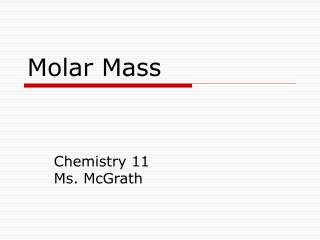DownloadDownload PresentationMolar Mass

# Molar Mass

Télécharger la présentation## Molar Mass

- - - - - - - - - - - - - - - - - - - - - - - - - - - E N D - - - - - - - - - - - - - - - - - - - - - - - - - - -
##### Presentation Transcript

1. Molar Mass Chemistry 11 Ms. McGrath

2. Molar Mass Molar mass (M) is the mass of one mole (6.02 x 1023 particles) of a substance numerically equal to the element’s average atomic mass (u). It is expressed in g / mol.

3. Molar Mass Molar masses of common chemical compounds that you might find in the chemistry laboratory can range between 18 grams/mole for compounds like water to hundreds of grams per mole for more complex chemical compounds.

4. Molar Mass The lightest possible chemical that one can have under normal conditions is hydrogen gas, or H2. There is no limit to how heavy a chemical compound can be - it is not uncommon for macromolecules (large organic or bioorganic compounds such as DNA) to weigh thousands of grams per mole.

5. Molar MassHow do I find Molar Mass? The molar mass of elements is found by looking at the atomic mass of the element on the periodic table. For example, if you want to find the molar mass of carbon, you would find the atomic mass of carbon on the periodic table, and this is equal to the molar mass in grams per mole. So, carbon has a molar mass of 12.01 grams per mole.

6. Molar MassHow do I find Molar Mass? There are a few exceptions to this rule. • In the case of H, N, O, F, Cl, Br, and I, the element is diatomic, meaning that each molecule of the element has two atoms of that element stuck together. • P is normally found in clumps of four atoms, and S is found in clumps of eight atoms. Still, aside from the exceptions above, all elements have the same molar mass as the atomic masses on the periodic table.

7. Molar MassHow do I find Molar Mass? Molar masses of chemical compounds are equal to the sums of the molar masses of all the atoms in one molecule of that compound. ex: BeO MBeO = 9.01 g/mol + 16.00 g/mol = 25.01 g/mol

8. Molar MassHow do I find Molar Mass? ex: Ca3(PO4)2 MCa x 3 = (40.08 g/mol) x 3 = 120.24 g/mol MP x 2 = (30.97 g/mol) x 2 = 61.94 g/mol MO x 8 = (16.00 g/mol) x 8 = 128.00 g/mol MCa3(PO4)2 = 120.24 g/mol + 61.94 g/mol + 128.00 g/mol = 310.18 g/mol

9. Molar MassPractice - Molar Mass Page 57 #16, 17, 18, 19

10. Molar MassConverting from Moles to Mass Mass = Number of moles x Molar Mass m = n x M

11. Molar MassConverting from Moles to Mass A flask contains 0.750 mol of carbon dioxide gas, CO2. What mass of carbon dioxide gas is in this sample? m = n x M MCO2 = 2 x (16.00 g/mol) + 12.01 g/mol = 44.01 g / mol m = n x M = (0.750 mol) x (44.01 g/mol) = 33.0 g

12. Molar MassPractice - Converting from Moles to Mass Page 59 #20, 21, 22, 23

13. Molar MassConverting from Mass to Moles Mass = Number of moles x Molar Mass m = n x M